# Analyze the least squares adjustment results

Adjustment results are stored in the following adjustment feature classes:

• AdjustmentLines—Stores and displays adjusted and statistical data for parcel lines, geodetic latitudes, and geodetic longitudes.
• AdjustmentPoints—Stores and displays adjusted and statistical data for parcel fabric points.
• AdjustmentVectors—Stores and displays the shifts between parcel fabric points and their adjusted points.

Adjustment feature classes are added to the map after the Analyze By Parcel Least Squares Adjustment tool has been executed.

## Analyze adjustment lines

The Adjustment Lines layer displays the following adjusted measurement subtypes:

• Distance—Stores information about the adjusted distances of the input parcel lines.
• Direction Set—Stores information about the adjusted direction sets of the input parcel lines. The foresight line in the direction set is the adjusted direction for the line.
• Geodetic Latitude—The x-coordinate of a weighted or constrained point converted to geodetic latitude.
• Geodetic Longitude—The y-coordinate of a weighted or constrained point converted to geodetic longitude.

### Check for outliers in dimensions

Check the Adjustment Lines layer for outliers in directions and distances. Outliers are lines with dimensions that are not consistent with the measurement network and indicate potential blunders or mistakes.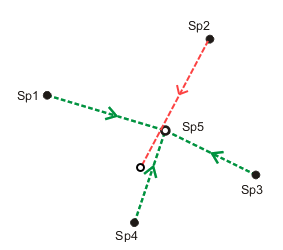In the Adjustment Lines layer, lines with outlier dimensions are highlighted in pink for outlier distances and yellow for outlier dimensions. The larger the Standardized Student's t Statistic attribute value for the dimension, the thicker the line symbology. Dimensions with larger Standardized Student's t Statistic attributes are deviating more than expected from the best-fit solution computed by the least squares adjustment.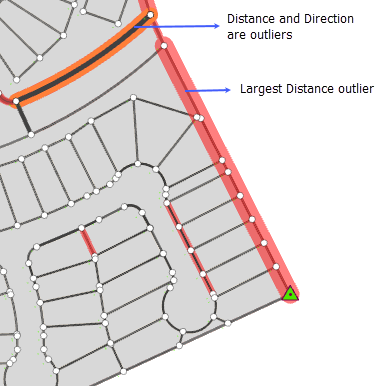A dimension is flagged as an outlier when the measurement correction between the original dimension and adjusted dimension of the line fails statistical testing against the Normal distribution at the 95 percent confidence level. A 95 percent confidence level means that 95 percent of all measurement corrections for the adjusted network are expected to be within 1.96 deviations from the mean (average) value. See a description of the Normal distribution in the section below.

Dimensions with Standardized Normal Statistic attributes that exceed +-1.96 are flagged as outliers (Outlier field is set to Yes).

Outlier dimensions should be addressed before proceeding with any further analysis.

### Check for geodetic latitude and geodetic longitude outliers

In a weighted least squares analysis, the x,y coordinates of control points (weighted and constrained) are converted to and input as geodetic latitude and geodetic longitude measurements into the DynAdjust least squares adjustment engine. The coordinate values are converted into latitudes and longitudes with associated standard deviations that are obtained from the XY Accuracy field on the parcel fabric Points feature class.

If the latitude and longitude values of the constrained or weighted points shift in the adjustment, the shifts are output as separate measurements with statistical information to the AdjustmentLines feature class.

In the Adjustment Lines layer, latitude and longitude corrections are displayed in the Geodetic Latitude and Geodetic sublayers using boxes that are drawn around the constrained or weighted points. Outlier corrections are displayed in red, and the larger the Standardized Student's t Statistic attribute value for the correction, the thicker the line symbology is.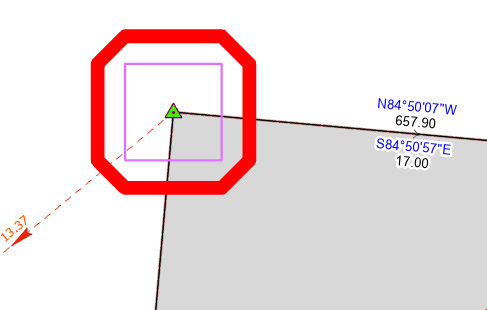##### Tip:
You can select the boxes to view the measurement and statistical information of the coordinate shift.

A latitude or longitude correction is flagged as an outlier when it fails statistical testing against the Normal distribution at the 95 percent confidence level. A 95 percent confidence level means that 95 percent of all measurement corrections for the adjusted network are expected to be within 1.96 deviations from the mean (average) value. See a description of the Normal distribution in the section below.

Latitude and longitude corrections with Standardized Normal Statistic attributes that exceed +-1.96 are flagged as outliers (Outlier field is set to Yes).

Follow these suggestions for addressing and reducing the outliers:

• Fix the largest outlier dimension first and rerun the least squares analysis. This is the line that has the thickest highlighted line symbology and has the largest measurement correction in the Measurement Correction field. Fixing the largest outlier often resolves any other outlier lines that are connected to it.
• To fix an outlier dimension, check the outlier dimension against the line dimensions on the record. If the dimensions do not match, select the parcel line, open the Attributes pane, and edit the dimension to match the recorded dimensions.
• If the record is not available to check the outlier dimension, exclude the line and rerun the least squares analysis (use a line selection as the input and leave the line unselected). If the analysis passes with no outliers, the excluded line most likely is erroneous and should remain excluded or should be corrected.
• If the dimensions do match the record, exclude the outlier line and rerun the least squares analysis. In some cases, reliable dimensions can be connected to a point that is connected to another line that contains the erroneous measurement.
• If a weighted or a constrained point is flagged as an outlier and there are no other dimension outliers in the network, check the coordinates of the point, as they could be incorrect.

### Evaluate unreliable dimensions

Measurement corrections are also tested against the Standardized Student's t distribution at a 95 percent confidence level. The larger the Standardized Student's t Statistic attribute value for the dimension, the thicker the gray Standard line symbology is in the Adjustment Lines layer.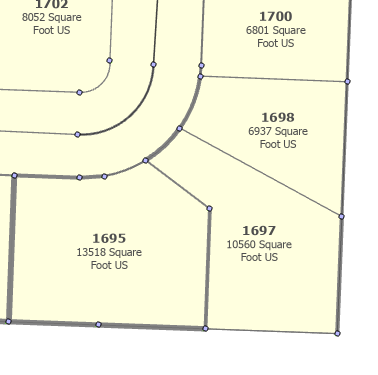Dimensions with larger Standardized Student's t Statistic attributes are deviating more than expected from the best-fit solution computed by the least squares adjustment. Dimensions that are not flagged as outliers (Standardized Normal Statistic does not exceed +-1.96) but have larger Standardized Student's t Statistic attributes are potentially unreliable and should be investigated further.

See more information about the Student's t distribution in the section below.

### Recommendations for detecting and reducing the occurrence of outliers

Initially, run a least squares analysis on parcels from the same record. These measurements will have the same characteristics, as they were most likely taken from the same measuring device. Analyze one record at a time. Once the record adjustments are passing without outliers, a larger adjustment can be performed on multiple adjacent records. By keeping adjustments separated by record, any outliers in one record will not distort and affect the measurements in another record, making the true outliers harder to detect.

## Analyze adjustment points

The adjustment points layer displays the adjusted locations of the parcel fabric points. When the results of a weighted least squares analysis are applied to the parcel fabric, parcel points will shift to their adjusted locations. When analyzing the reliability of adjusted point coordinates, the error ellipses and the positional uncertainty of points should be evaluated.

### Error ellipses

Adjustment point features are uniquely symbolized with error ellipses using values in the Error Ellipse Semi Major and Error Ellipse Semi Minor fields.

Error ellipses are used to represent the positional uncertainty of the x,y coordinates of the adjusted point. An error ellipse around a point indicates the possible variances of the x- and y-coordinates at a 95 percent confidence level. The 95 percent confidence level means that 95 percent of the estimated x- and y-values of the point are expected to lie within the circular region defined by the error ellipse when coordinate deviations are tested against the Normal and Student's t distribution. The most probable values of the x- and y-coordinates coincide with the center of the ellipse.

For more information on the Normal and Student's t distribution, see the sections below.

The smaller and rounder the error ellipse, the less variance there is in the estimation of the x- and y-coordinates and the more reliable the adjusted coordinates are for the point. For example, in the image below, the error ellipse is small, indicating that there is not much variance (uncertainty) in the x,y coordinates. The shape of the ellipse furthermore indicates that there is not much more deviation in either the x- or y-coordinate when compared with each other.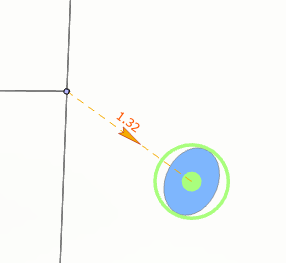The larger the error ellipse, the more variance and uncertainly there is in the estimated x,y coordinates. For example, in the image below, there is a large variance and a large degree of uncertainty in the y-coordinate value. There is less uncertainty in the estimate of the x-coordinate of the point.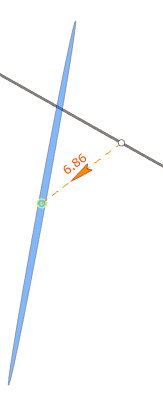Large error ellipses around adjusted points could be result of the following factors:

• Outliers or unreliable dimensions in the parcel line network are causing distortions and large degrees of uncertainty in the adjusted coordinates. Address outliers as described above and rerun the analysis.
• There are not enough constrained or weighted control points in the network that was analyzed. Sparsely distributed control points can cause large degrees of uncertainly in adjusted coordinates. Control points should be evenly distributed in the network being analyzed for the best estimates of coordinates.
• The network is poorly connected in the area surrounding the point. This can occur when blocks of parcels are not connected across rights-of-way. Add connection lines to increase network redundancy in this area of the parcel network.

### Positional uncertainty

The estimated positional uncertainty for each adjusted point is stored in the XY Uncertainty field in the AdjustmentPoints feature class. The positional uncertainty value is derived from the semimajor and semiminor axes of the error ellipse and is computed at a 95 percent confidence level. Points with positional uncertainties that exceed +-1.96 should be investigated further.

See a description of the Normal distribution below for more information.

To improve the positional uncertainty value or accuracy of an adjusted point, additional control points or more measurement information in this area of the network may be needed. Outliers and unreliable dimensions can also contribute to high positional uncertainty values for a point.

## Normal distribution

The Normal distribution, also known as the bell curve, models how measurements of a quantity are distributed. The Normal distribution expects most measurements to cluster around the mean value, which is the central peak of the curve. The probability that measurements will deviate from the mean decreases symmetrically from both sides of the curve. The larger the deviation, the smaller the expected probability is.

In a Normal distribution, 95 percent of the area underneath the curve lies within approximately 1.96 standard deviations of the mean.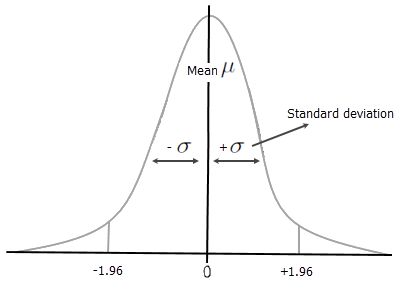## Student's t distribution

When adjusting measurement networks where the number of measurements is limited (small networks), more reliable statistical testing can be gained from using the Student's t distribution. Testing against the Student's t distribution is also better suited to measurement networks where there is low confidence in the a priori estimates (the assumed standard deviations of the measurements).

The Student's t distribution models a best estimate of how measurements of a quantity are distributed where the standard deviations are not known. The shape of the curve is symmetric and bell-shaped like the Normal distribution but may have a shorter, narrower peak and wider tapering of the tails, meaning that more values that deviate far from the mean are expected. The Student's t distribution has a lower tendency to mark deviations in measurements as outliers.